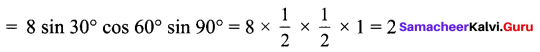# Samacheer Kalvi 9th Maths Solutions Chapter 6 Trigonometry Ex 6.2

## Tamilnadu Samacheer Kalvi 9th Maths Solutions Chapter 6 Trigonometry Ex 6.2

Question 1.
Verify the following equalities:
(i) sin2 60° + cos2 60° = 1
(ii) 1 + tan2 30° = sec2 30°
(iii) cos 90° = 1 – 2 sin2 45° = 2 cos2 45° – 1
(iv) sin 30° cos 60° + cos 30° sin 60° = sin 90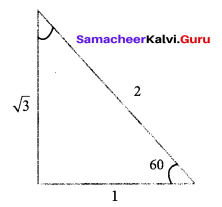Solution: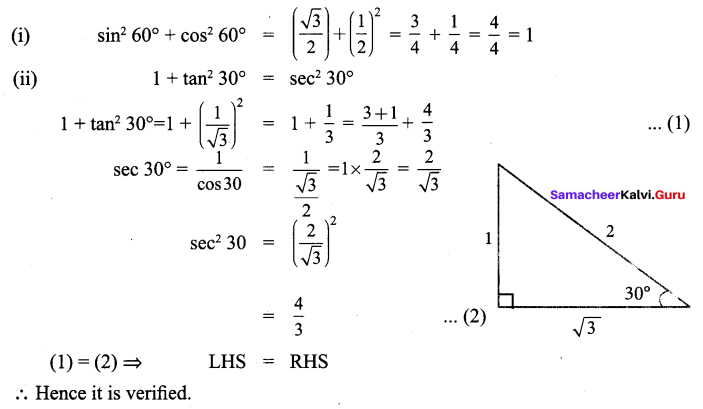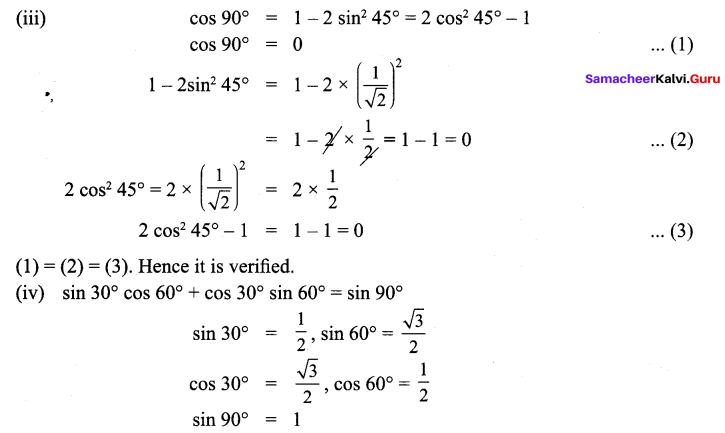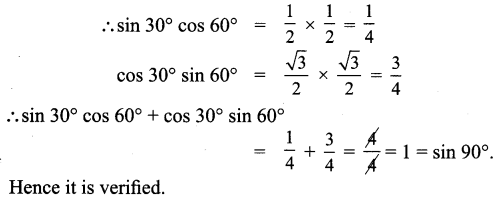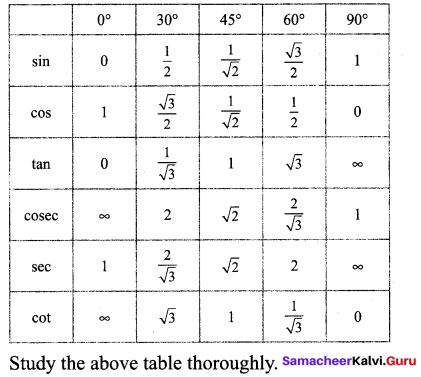Question 2.
Find the value of the following: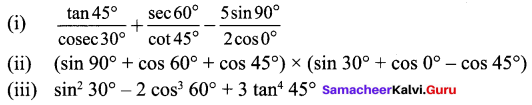Solution: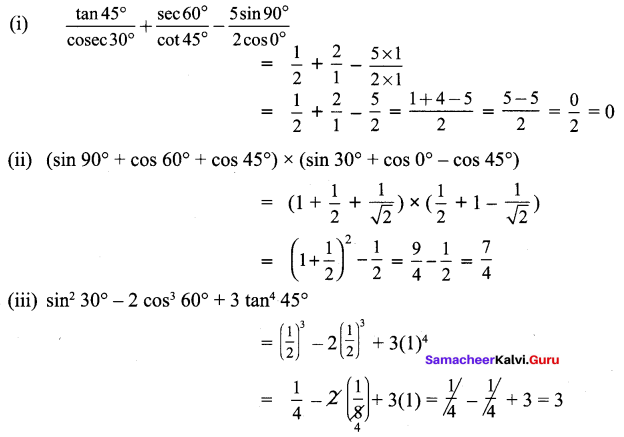Question 3.
Verify cos 3A = 4 cos3A – 3 cos A, when A = 30°
Solution:
L.H.S = cos 3A = cos 3(30°) = cos 90° = 0
R.H.S = 4 cos3A – 3 cos A = 4 cos330° – cos 30°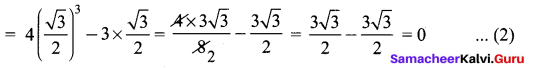(1) = (2). Hence it is verified.

Question 4.
Find the value of 8 sin 2x cos 4x sin 6x, when x = 15°
Solution:
8 sin 2(15°).cos 4(15°).sin 6(15°)